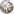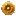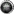Adds a game currency amount with game icons to a wiki page. Can also use {{currency}} instead of {{cost}} to the same effect, if wording makes more sense in context.

Usage
{{cost|<plat>|<gold>|<silver>|<copper>}}
1= [number] (Optional) - platinum amount
2= [number] (Optional) - gold amount
3= [number] (Optional) - silver amount
4= [number] (Optional) - copper amount
ren= [number] (Optional) - Renown amount
pre= [number] (Optional) - Prestige amount
eg= [number] (Optional) - Elder Gem amount
cv= [number] (Optional) - Crafting Voucher amount
Examples

Platinum: 1`{{cost|1}}`

Gold: 1`{{cost||1}}`

Silver: 1`{{cost|||1}}`

Copper: 1`{{cost||||1}}`

Platinum, Gold, Silver, Copper: 1111`{{cost|1|1|1|1}}`
`{{cost|ren=1}}`
`{{cost|pre=1}}`
`{{cost|eg=1}}`
`{{cost|cv=1}}`